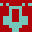# euler303

#### Project Euler #303

```\ Euler Problem 303: http://projecteuler.net/problem=303

\ For a positive integer n, define f(n) as the least positive multiple
\ of n that, written in base 10, uses only digits <= 2.

\ Thus f(2)=2, f(3)=12, f(7)=21, f(42)=210, f(89)=1121222.

\ find sum(1..10000,f(n)/n)

\ Solution: generate all f(n) of the desired form ("cool numbers"),
\ starting with the smallest, and see if any of the remaining n divide
\ it.

\ This program conforms to Forth-94

10000 constant limit

limit 998 > 1 cells 8 < and throw \ needs a 64-bit system to run for limit>998

: gen-ns ( n -- )
1+ 1 ?do i , loop ;

create ns limit gen-ns
variable end-ns
variable sum 0 sum !

: desired-form ( u1 -- ud2 )
\ u2 is the u1th cool number
3 base ! 0 <# #s #>
decimal 0 0 2swap >number 2drop ;

: remove-n ( addr -- )
\ remove the number at addr from ns
end-ns @ -1 cells + 2dup u< if ( addr addr1 )
2dup @ swap !
then
end-ns ! drop ;

: sift ( ud -- )
\ see if u is dividable by any of the ns; if so, add u/n to sum
\ and remove n from ns
ns begin ( ud addr )
>r 2dup r@ @ um/mod swap 0= if
sum +! r> dup remove-n
else
drop r> cell+
then
dup end-ns @ = until
drop 2drop ;

: euler303 ( u1 -- u2 )
cells ns + end-ns !
1 begin ( i )
dup desired-form sift 1+
end-ns @ ns = until
sum @ ;

limit euler303 .

```

## by AntonErtl